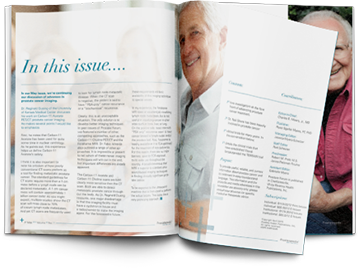# Prostate Forum - Vol. 14, Issue Number 6

\$ 9.00

In this issue of Prostate Forum, Dr. Snuffy Myers talks about: Bone Mets & PSA <10 ng="" ml="" intermittent="" continuous="" adt="" maha="" hussain="" s="" recent="" findings="" rt="" via="" imrt="" bowel="" problems="" supplements="" egg="" whites="" verus="" whole="" eggs="" depo-lupron="" diabetes="" ibs="" drugs="" brain="" function="" peanut="" butter="" 3="" t="" mri="" psa-only="" recurrence="" after="" irradiating="" lymph="" nodes="" testosterone="" replacement="" as="" and="" ip6="" span="">

Volume 14, Issue 6